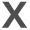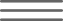## Compare Aircraft Costs Using NPV

When you consider a change in aircraft usage or aircraft ownership, you should evaluate the total cost method for using the aircraft as well as how a specific aircraft “A” compares to aircraft “B”.  Do you own, lease, or contract for the usage of the aircraft?  How does the costs of using aircraft “A” compare to aircraft “B”?  Which usage methods and aircraft is the better deal from a financial viewpoint?

Please notice that no qualifiers have been placed on the usage method, intended usage, or specific expense category of either aircraft “A” or “B”.  It might not even be an apples-to-apples comparison.  You might already own “A” and want to compare the cost of keeping “A” to trading it for “B”.  You might be comparing leasing, owning or contracting aircraft usage of several different aircraft models.  Your interest is strictly financial.  From a big picture standpoint, aircraft “A” and “B” could be investment “A” and “B”.  Which investment is the better deal from a financial viewpoint?  How do you make this financial comparison and answer this question?  Please notice that the features and benefits of the aircraft usage methods or aircraft types is a totally different question that you should also be evaluating.  This paper only discusses the financial consequences of an investment.

Please realize the importance of addressing these concerns today due to our current economic situation.  Start planning now to determine the best financial decisions for your situation.  When Congress passes the new tax laws, you can further evaluate your financial plan.  Using NPV to evaluate your financial alternatives and specific aircraft models could save you significant money.  If you aren’t familiar with NPV, do a little homework now so you have time to study and understand the impact NPV can have on any of your financial decisions.

WHAT IS NPV?

Use Net Present Value (NPV) to evaluate and compare any situation “A” and “B” financially.  First, your rocket-science hat is not required to understand this concept.  Please be patient and read carefully as only your common sense is required.  When money is spent today that produces a cash flow (receipts or distributions) in the future, you can use NPV to calculate the value of this cash flow in today’s dollars.  All investment decisions center on calculating today’s value of a future cash flow.  This is more complicated than the simple addition and subtraction of the cash receipts because a dollar today is worth more than a future dollar.  You must consider the time value of money.  Performing a Net Present Value (NPV) analysis will provide you an answer on the current dollar value of your investment.  You can use before or after tax cash flows to calculate today’s dollar value on any investment.  Using after-tax cash flows provides the advantage of considering all tax benefits in your investment decision.

The Net Present Value (NPV) is a single dollar value that is calculated from a cash flow over a period of time which has been adjusted for the time value of money.  The NPV of an investment is the difference between the sums of the cash flows (adjusted for the time value of money) produced by the investment less the amount initially invested.  To adjust the cash flows to reflect the time value of money requires that each cash flow value must be adjusted for the time it was received (or disbursed) and the interest rate used to calculate the present dollar value.  The interest rate used in the calculation is known as the discount rate.  The discount rate used is usually your current cost of borrowing or the percentage return you could achieve with a safe investment.  The single dollar value of the total adjusted cash flow not including the initial investment is known as the Present Value (PV).  Therefore:

Net Present Value (NPV) = Present Value (PV) less Initial Investment.

As an example, assume \$100,000 is the initial investment in an aircraft.  This aircraft investment produces a cash flow of \$25,000 a year for 5 years.  The total cash flow received over the five-year period is \$125,000.  The aircraft is sold at the end of Year 5 for \$70,000.  Assume an interest rate (discount rate) of 10%.  The adjusted cash flow for the time the payment was received (assumed here to be at the end of each year) and the 10% interest rate produces a Present Value (PV) of \$138,234.  See “Net Present Value Example” table and “HOW TO COMPUTE NPV” section below.  The actual financial PV formula was used to calculate the “Adjustment Factor” in the table.  However, it is much easier to use a financial calculator that contains the NPV function.

NPV = \$38,234 = \$138,234 (PV) less \$100,000 (Initial Aircraft Investment)

By definition; if the NPV=0, then the investment return is equal to the discount rate.  If the NPV is greater than zero then the investment return has exceeded the discount rate.  If the NPV is less than zero then the investment return is less than the discount rate.  In this example, the NPV is a positive \$38,324.  If you make this initial investment of \$100,000, you will earn \$38,324 more than 10%.  A discount rate of 21.05% will produce a NPV=Zero, which means the investment return was 21.05%.

HOW DO YOU COMPUTE NPV?

Use a financial calculator or use the NPV financial function in Microsoft Excel to make the calculation.  NOTE:  Excel’s language about discounted cash flows differs from the standard finance nomenclature.  Excel uses the letters NPV to denote the Present Value (not the Net Present Value) of a cash flow.  To calculate the finance Net Present Value of an investment using Excel, calculate the Present Value using the Excel NPV financial function then subtract the amount of the initial investment. For your information, the IRR financial function in Microsoft Excel was used to calculate the investment return of 21.05% noted above.

The AircraftCostAnalysis program can be used to produce NPV analysis for any type aircraft, ownership method, lease or purchase, rent or contract situation.  Do the math before you invest.  Obtain the details at www.AircraftCostAnalysis.com.